Abstract

In order to study the effect of the limit equilibrium zone energy in front of the heading face on the volume of coal and gas outburst, the concept of coal-gas mixed matrix was put forward, then the width of the limit equilibrium zone was calculated, and thus the energy transformation mathematical model of the limit equilibrium zone was established through theoretical analysis and mathematical derivation. In addition, the energy density calculation formula of the limit equilibrium zone was derived and then the intensity prediction formula of coal and gas outburst was derived combining elastic energy of coal body, expansion energy of gas, crushing work and throwing work of coal body. The results showed that the energy in the limit equilibrium zone has a certain effect on the volume of coal and gas outburst and the intensity prediction formula has high accuracy in Yangou Coal Mine. These conclusions have certain guiding significance for energy calculation and intensity prediction of coal and gas outburst.

Keywords

Coal and gas outburst, limit equilibrium zone, coal-gas mixed matrix, energy density, intensity prediction

1 Introduction

BP's latest 2019 World Energy Outlook report showed that coal demand of China will account for 39% of global coal demand by 2040 although the coal consumption in total energy consumption in China will fall from 60% in 2017 to 35% in 2040. Therefore, coal will continue to play an important part in energy consumption. The depth of coal mining will increase in the wake of the gradual reduction of shallow coal resources, and the possibility of coal and gas outburst (CGO) will rise . Many researchers were devoted to relevant study on CGO. B.B. Hodort  has proposed the energy theory to explain the cause of CGO from the perspective of energy, and Liu et al.  analyzed that from the perspective of mechanics, Nie et al.  discussed the mechanism of CGO caused by vibration. Moreover, a stress-seepage-damage coupling model was established to investigate the size scale of CGO . Some new technologies and experiments were used for further study, such as acoustic emission and microseismic monitoring [6-8], numerical simulation [9-11], three-dimensional testing system  and simulation experiment [13-14].

There are various factors affecting CGO, Zhai et al.  found that the factors include geological structure, mining depth, seam thickness, the lithology of roof and floor, and the mode of operation. In addition, the widespread occurrence of tectonic coal and soluble organic matters play a crucial role in outbursts [16, 17]. Temperature is of the least importance in the outburst intensity of coal and gas among gas pressure, the thickness of coal and the temperature . Human errors and the lack of safety knowledge and awareness are the main causes of CGO from the perspective of human .

The energy of CGO is of great importance because it determines the intensity of CGO. A method was provided to calculate gas expansion energy based on the effect of coal damage and the environmental pressure change for desorption . More gas and outburst energy were demonstrated in the Gas-enriched areas, which can cause an outburst easily . And some researchers found the gas expansion energy is higher than the elastic energy of coal .

Effective prediction can reduce the probability of CGO. A new method for predicting CGO was proposed based on coal mass strength, gas pressure, and in situ stress . Potential coal emissions can be predicted by measuring the initial expansion energy of coal lumps in the prone areas and the thickness of soft coal. The relationship between the initial expansion energy of released gas and the initial volume of released gas can reflect the risk of coal outburst . A new comprehensive index was adopted to predict coal-rock dynamic disaster hazards . Jiang et al.  proposed a method to identify the coal seams of CGO and the evaluation indexes. And in the study of Aziz et al. , a mine safety management system was established to decrease the incidence of CGO. In addition, some theories and new technologies were used to predict CGO, such as BP neural network , Fisher criterion , support vector machine , Bayesian network , gray theory  and fault tree .Furthermore, some researchers demonstrated that uniform fracturing , hydraulic slotting technique [35, 36] and mining protective layers [37-42] can effectively reduce the risk of CGO.

The researches above on CGO mainly focused on the mechanism, influencing factors, prediction and prevention measures. However, the energy of the limit equilibrium zone has been rarely concerned although some researchers have done a lot of research on the gas energy and expansion energy of CGO. This study established a mathematical model to calculate the energy density of the limit equilibrium zone and thus the total energy of it. In addition, the intensity prediction formula of CGO was derived combining elastic energy of coal body, expansion energy of gas, crushing work and throwing work of coal body, and the accuracy has been verified in Yangou Coal Mine. These results will have guiding significance for the energy calculation and intensity prediction of CGO.

# 2 Limit equilibrium zone energy density

The occurrence probability of CGO in the heading face is higher than other work places according to statistics. Therefore, the coal and rock mass in front of the heading face is the key point to the research of CGO. The overburden load of the goaf is transferred to the front of the heading face during the normal advancing process of the heading face. Hence, the vertical pressure of the coal rock mass in front of the heading face within a certain range is larger than the original vertical pressure. Under the action of abutment pressure, part of the coal body undergoes strength failure, and it continues to carry with the pressure after failure, and it is called the limit equilibrium zone.

The energy stored in the limit equilibrium zone is transformed from elastic energy and gas expansion energy, and there is no existing formula to calculate it directly. In order to calculate the energy of the limit equilibrium zone, the mixture of coal body and gas in this zone can be considered as a kind of even material, which is called coal-gas mixed matrix. Then the energy density calculation formula of the limit equilibrium zone can be obtained, based on the energy conservation principle during the process of the elastic zone transforms into the limit equilibrium zone. The derivations are based on the following hypotheses:

a. Methane is ideal gas and desorbs in an instant;

b. Coal-gas mixed matrix is an even material;

c. Coal and gas outburst process is an adiabatic process.

## 2.1 Limit equilibrium zone width

The pressure around the heading face will be redistributed because of the effect of roadway excavation, as shown in Figure 1. Taking the section view along the advancing direction of the heading face, the pressure distribution and the zone division can be shown clearly in Figure 2, the zones include limit equilibrium zone and elastic zone .

At the boundary between the limit equilibrium zone and the elastic zone, the pressures satisfy,

where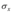is the horizontal pressure, kPa;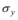is the vertical pressure, kPa;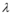is the lateral pressure coefficient; K is the pressure concentration factor;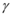is the bulk density of the overlying strata, N/m3; H is the burial depth of coal seam, m.

Fig. 1 Pressure distribution around heading face

The pressure of coal body in the limit equilibrium zone satisfies the limit equilibrium condition, and its differential equilibrium equations are:

where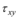is the shear pressure, kPa;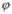is the internal friction angle of coal seam, °; c is the coal seam cohesion, kPa.

Combine equations (1) and (2), we can obtain

Fig. 2 Pressure distribution in front of heading face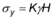. Then we can obtain the limit equilibrium zone width r0.

where m is the thickness of the coal seam, m.

2.2 Transition zone elastic energy

In the original rock pressure zone, the coal body satisfies the characteristics of elastomer, and the stored energy can be calculated according to the elastic energy calculation formula. The elastic energy in per unit volume of coal body is:

where E is the coal seam modulus of elasticity, MPa;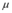is the coal seam Poisson's ratio;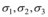are main pressures, MPa.

Assuming that the section of tunneling roadway is circular and its radius is r0. If the tunneling distance is 1 m, the elastic zone with corresponding length will transform into limit equilibrium zone, as shown in Figure 3. The mathematical model and the orthogonal coordinate system was established as shown in Figure 4, the tunneling direction of the roadway as the positive x-axis and the vertical direction of the heading face as the positive y-axis. The shadow part is the research zone where the energy transformed from elastic zone to limit equilibrium zone, and the volume is V1.

Fig. 3 Three-dimensional structure model of energy transition zone
Fig. 4 Mathematical model of energy transition zone. Note: r-Roadway radius, m; r0-Limit equilibrium zone radius, m

where y is the distance from the center of the roadway, m; f1(y), f2(y) are the before and after transformation curve equations of the interface between the limit equilibrium zone and the elastic zone respectively.

f1(y), f2(y) satisfy

The energy stored in the limit equilibrium zone that transformed from the elastic zone is Ee:

2.3 Transition zone gas energy

The existing state of gas in coal body includes free state and adsorbed state. If there is no disturbance, the energy of gas is stored in coal body in the form of internal energy. The calculation formula is as follows:

where Eg is the gas internal energy, J; i is the freedom degree of gas; n is the quantity of matter, mol; R is the gas constant, J/(mol·K); T is the absolute temperature, K.

Assuming that the gas in the coal body is an ideal gas and satisfies the ideal gas state equation, then

where P is the gas pressure, MPa; Vg is the volume of gas, m3.

In the energy transition zone,

where q is the gas content of per ton coal, m3/t.

Substituting equations (10) and (11) into equation (9) yields

As CH4 is a polyatomic gas, i takes 6. Substituting it into equation (12) then

2.4 Transition zone energy consumption

When coal body transforms from elastic state to limit equilibrium state, coal body will generate cracks, which will consume the energy of coal-gas mixed matrix. Because the number and length of cracks cannot be directly measured, it is difficult to obtain a formula through theoretical derivation to calculate the energy consumption, thus the formula can be obtained only from experimental tests .

where Eb is the energy consumed by coal body crushing, J; f is the consistent coefficient of coal body;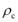is the coal body density, kg/m3; Yp1is the percentage of the quality of coal samples with particle size less than 0.2 mm accounts for the total coal samples.

The displacement is assumed not to change when the coal body transforms from elastic state to limit equilibrium state, and its moving work is not considered here.

2.5 Limit equilibrium zone energy density

When elastic zone with volume of V1 becomes limit equilibrium zone, then the corresponding energy is stored in the limit equilibrium zone, which can be set as EL.

According to the energy dynamic balance, we can obtain:

Substituting equations (8), (13) and (14) into (15) then

Assuming that the energy density of the limit equilibrium zone is e2, then

3 CGO intensity prediction

3.1 CGO supporting energy

CGO supporting energies contain: free gas expansion energy, adsorbed gas expansion energy, elastic energy, and limit equilibrium zone energy. Assuming that the CGO volume is V2, the energies are as follows respectively.

#### (1) Free gas expansion energy 

where W1 is the free gas expansion energy, J; Vg is the free gas content, m3; a is the proportion of free gas participating in expansion work, J; Tgis the absolute temperature after gas expansion, K; p0 is the initial gas pressure, MPa; p1 is the pressure after gas expansion, MPa; Vmis the molar volume of gas in standard state, m3/mol; s is the variable process index;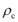is the coal body density, kg/m3.

where b is the percentage of free gas in total gas.

Substituting equation (19) into (18), we can get

(2) Adsorbed gas desorption expansion energy

where W2 is the desorption expansion energy of adsorbed gas, J; Va is the gas volume in adsorbed gas participating in work, m3; T0 is the gas initial absolute temperature, K.

where d is the percentage of adsorbed gas participating in expansion work.

Substituting equation (22) into (21), then we can get

(3) Elastic energy

When the CGO volume is V2, the corresponding elastic energy is Ee.

(4) Limit equilibrium zone energy

When the CGO volume is V2, the limit equilibrium zone is V1 and the corresponding limit equilibrium zone energy is EL.

3.2. CGO Energy consumption

The main energy consumption of CGO contain the crushing work and throwing work of coal body, as well as the energy consumption of friction and noise. In view of the less energy consumption of friction and noise, this paper will not consider it for the convenience of calculation.

(1) Crushing Work

where W3 is the crushing work corresponding to CGO volume V2, J.

(2) Throwing Work

The throwing work of the coal body is the corresponding kinetic energy when the broken coal is thrown out,

where W4 is the throwing work corresponding to CGO volume V2, J; v is the throwing speed of broken coal, m/s.

3.3. CGO intensity prediction

According to the law of conservation of energy, we can obtain

That is

 (29)

Deform equation (29), we can set

 (30)

where B is the CGO intensity, t.

4. Model validation

4.1. Variables and parameters

The variables and parameters of CGO in Yangou coal mines are shown in Table 1 by querying the relevant data and references .

Table 1 Variables and parameters of Yangou Coal Mine
 Variable Parameter Value Unit B Intensity of coal and gas outburst 2000 t Lateral pressure coefficient 0.54 K Pressure concentration factor 2.1 Bulk density of the overlying strata 3500 kg/m3 H Burial depth of coal seam 460m m m Thickness of the coal seam 4m m Internal friction angle of coal seam 25 ° c Coal cohesion of coal seam 0.86 MPa Poisson's ratio of coal seam 0.35 E Coal seam modulus of elasticity 3600 MPa Main pressure 18.34 MPa 11.58 MPa 10.62 MPa R Gas constant 8.314 J/(mol·K) q Gas content of per ton coal 11.25 m3/t f Consistent coefficient of coal body 0.24 Coal body density 1300 m³/t Yp1 Percentage of the quality of coal samples with particle size less than 0.2mm accounts for the total coal samples 40 Tg Free gas content 298 K T0 Gas initial absolute temperature 296 K b Percentage of free gas in total gas 0.80 a Percentage of free gas participating in expansion work 0.8 d Percentage of adsorbed gas participating in expansion work 0.32 p0 Initial gas pressure 1.12 MPa p1 Pressure after gas expansion 0.2 MPa v Speed of coal outburst 30 m/s

4.2. Result analysis

The energy of CGO is the key factor to determine the outburst intensity. In this paper, the energy in the limit equilibrium zone was considered. Through theoretical derivation, a method for accurately calculating the energy of CGO was proposed. Then, according to the actual situation of Yangou coal mine, the method was verified. The specific analysis process is as follows.

According to equations (20), (23), (24), (25), (26), (27), the parameters containing the width and energy of limit equilibrium zone, elastic energy, free gas expansion energy, adsorbed gas expansion energy, crushing work and throwing work were calculated, thus the CGO intensity of Yangou Coal Mine can be obtained. And the each energy of CGO is shown respectively in Tabal 2.

Table 2 Energy calculation
 Variable Parameter Value Unit EL Energy of the limit equilibrium zone 8.326×104 J Ee Elastic energy 1.217×102 J W1 Expansion energy of free gas 6.019×106 J W2 Expansion energy of adsorbed gas 5.472×105 J W3 Crushing work 5.771×106 J W4 Throwing work 8.789×105 J

From Table 2, the limit equilibrium zone energy is 8.326×104 J, which accounts for 1.25% of the total energy, and the CGO volume corresponding to this energy is about 25 t. Moreover, the CGO prediction intensity is approximately 1953 t, and the error is 2.35% (lower than 5%) compared to the actual intensity 2000 t. Therefore, the accuracy of the mathematical model is reasonable in Yangou Coal Mine.

5. Conclusions

In this paper, we studied the effect of the limit equilibrium zone energy on the volume of CGO. The concept of coal-gas mixed matrix was put forward and the width of the limit equilibrium was calculated. Based on energy conservation law, a series of calculation and derivation were carried out to obtain the limit equilibrium zone energy density formula. Then the CGO intensity prediction model was established and verified. The results showed that in the total CGO volume of Yangou Coal Mine, 25 t was contributed by the limit equilibrium zone energy in front of the heading face. In addition, the accuracy of CGO intensity prediction was very high.

Acknowledgement

This work was supported by the National Natural Science Foundation of China (grant number 51374121) and the Liaoning Distinguished Professor Funding Project (grant number 551710007007).

References

 Xue S., Yuan L., Wang Y.,Xie J. umerical analyses of the major parameters affecting the initiation of outbursts of coal and gas. Rock Mech. Rock Eng., 47:1505-1510, 2014. https://doi.org/10.1007/s00603-013-0425-4
 Xодотвв. Coal and gas outburst. Song S., Wang Y., translation. China Industry Press: Beijing, China, 1966.
 Liu H., Lin B., Mou J.,Yang W. Mechanical evolution mechanism of coal and gas outburst. Rock Mech. Rock Eng., 52(5):1591-1597,2019. https://doi.org/10.1007/s00603-018-1546-6
 Nie B., Li X. Mechanism research on coal and gas outburst during vibration blasting. Safety Sci., 50(4): 741-744,2012. https://doi.org/10.1016/j.ssci.2011.08.041
 Fan, C., Li, S., Luo, M., Du, W.,Yang, Z. Coal and gas outburst dynamic system. Int. J. Min. Sci. Tech., 27(1): 49-55,2017. https://doi.org/10.1016/j.ijmst.2016.11.003
 Li, J., Hu, Q., Yu, M., Li, X., Hu, J.,Yang, H."Acoustic emission monitoring technology for coal and gas outburst. Energy Sci. Eng., 7(2), pp, 443-456,2019. https://doi.org/10.1002/ese3.289
 Li H., Feng Z., Zhao D.,Duan D. Simulation experiment and acoustic emission study on coal and gas outburst. Rock Mech. Rock Eng., 50(8): 2193-2205,2017. https://doi.org/10.1007/s00603-017-1221-3
 Lu C., Dou L., Zhang N., Xue J,Liu, G. Microseismic and acoustic emission effect on gas outburst hazard triggered by shock wave: a case study. Nat. hazards, 73(3):1715-1731,2014. https://doi.org/10.1007/s11069-014-1167-7
 Xue S., Wang Y., Xie J.,Wang G. A coupled approach to simulate initiation of outbursts of coal and gas—model development. Int. J. Coal Geol., 86(2-3): 222-230,2011. https://doi.org/10.1016/j.coal.2011.02.006
 An F., Cheng Y., Wang L.,Li W. A numerical model for outburst including the effect of adsorbed gas on coal deformation and mechanical properties. Comput. Geotech., 54: 222-231,2013. https://doi.org/10.1016/j.compgeo.2013.07.013
 Wang K., Zhou A., Zhang J.,Zhang P. Real-time numerical simulations and experimental research for the propagation characteristics of shock waves and gas flow during coal and gas outburst. Safety Sci., 50(4):835-841,2012. https://doi.org/10.1016/j.ssci.2011.08.024
 Yin G., Jiang C., Wang J., Xu J., Zhang D.,Huang G. A new experimental apparatus for coal and gas outburst simulation. Rock Mech. Rock Eng., 49(5):2005-2013,2016. https://doi.org/10.1007/s00603-015-0818-7
 Cao J., Sun H., Wang B., Dai L., Zhao B., Wen G.,Zhao X. A novel large-scale three-dimensional apparatus to study mechanisms of coal and gas outburst. Int. J. Rock Mech. Min. Sci., 118:52-62,2019. https://doi.org/10.1016/j.ijrmms.2019.04.002
 Hu Q., Zhang S., Wen G., Dai L.,Wang B. Coal-like material for coal and gas outburst simulation tests. Int. J. .Rock Mech. Min. Sci., 74:151-156,2015. https://doi.org/10.1016/j.ijrmms.2015.01.005
 Zhai C., Xiang X., Xu J.,Wu S. The characteristics and main influencing factors affecting coal and gas outbursts in Chinese Pingdingshan mining region. Nat. Hazards, 82(1):507-530,2016. https://doi.org/10.1007/s11069-016-2195-2
 Tu Q., Cheng Y., Ren T., Wang Z., Lin J.,Lei Y. Role of Tectonic Coal in Coal and Gas Outburst Behavior During Coal Mining. Rock Mech. Rock Eng., 52(11):4619-4635,2019. https://doi.org/10.1007/s00603-019-01846-0
 Yang Y., Sun J., Li Z., Li J., Zhang X., Liu L., Yan D.,Zhou Y. Influence of soluble organic matter on mechanical properties of coal and occurrence of coal and gas outburst. Powder Technol., 332:8-17,2018. https://doi.org/10.1016/j.powtec.2018.03.053
 Wang C., Yang S., Yang D., Li X.,Jiang C. Experimental analysis of the intensity and evolution of coal and gas outbursts. Fuel, 226:252-262,2018. https://doi.org/10.1016/j.fuel.2018.03.165
 Fu G., Xie X., Jia Q., Tong W.,Ge Y. Accidents analysis and prevention of coal and gas outburst: understanding human errors in accidents. Process Saf. Environ., 134:1-23,2020. https://doi.org/10.1016/j.psep.2019.11.026
 An F., Yuan Y., Chen X., Li Z.,Li L. Expansion energy of coal gas for the initiation of coal and gas outbursts. Fuel, 235:551-557,2019. https://doi.org/ 10.1016/j.fuel.2018.07.132
 Tu Q., Cheng Y., Guo P., Jiang J., Wang L.,Zhang R. Experimental study of coal and gas outbursts related to gas-enriched areas. Rock Mech. Rock Eng., 49(9):3769-3781,2016. https://doi.org/10.1007/s00603-016-0980-6
 Nie F., Wang H.,Qiu L. Research on the Disaster-Inducing Mechanism of Coal-Gas Outburst. Adv. Civ. Eng., 2020,2020. https://doi.org/10.1155/2020/1052618
 Dong G., Liang X.,Wang Q. A New Method for Predicting Coal and Gas Outbursts. Shock Vib., 2020,2020. https://doi.org/10.1155/2020/8867476
 Yang D., Chen Y., Tang J., Li X., Jiang C., Wang C.,Zhang C. Experimental research into the relationship between initial gas release and coal-gas outbursts. J. Nat. Gas Sci. Eng., 50:157-165,2018. https://doi.org/10.1016/j.jngse.2017.12.015
 Tang Z., Yang S., Zhai C.,Xu Q. Coal pores and fracture development during CBM drainage: their promoting effects on the propensity for coal and gas outbursts. J. Nat. Gas Sci. Eng., 51:9-17,2018. https://doi.org/10.1016/j.jngse.2018.01.003
 Jiang C., Xu L., Li X., Tang J., Chen Y., Tian S.,Liu H. Identification model and indicator of outburst-prone coal seams. Rock Mech. Rock Eng., 48(1):409-415,2015. https://doi.org/10.1007/s00603-014-0558-0
 Aziz N., Black D.,Ren T. Keynote paper mine gas drainage and outburst control in Australian underground coal mines. Procedia Eng., 26:84-92,2011. https://doi.org/10.1016/j.proeng.2011.11.2143
 Wu Y., Gao R.,Yang J. Prediction of coal and gas outburst: A method based on the BP neural network optimized by GASA. Process Saf. Environ., 133: 64-72,2020. https://doi.org/10.1016/j.psep.2019.10.002
 Li X., Jiang C., Tang J., Chen Y., Yang D.,Chen Z. A Fisher’s criterion-based linear discriminant analysis for predicting the critical values of coal and gas outbursts using the initial gas flow in a borehole. Math. Prob. Eng., 2017,2017. https://doi.org/10.1155/2017/7189803
 Yan Z., Yao K.,Yang Y. A novel adaptive differential evolution SVM model for predicting coal and gas outbursts. J. Differ. Equ. Appl., 23(1-2):238-248,2017. https://doi.org/10.1080/10236198.2016.1214725
 Nie W., Yang H., Zhang H., Geng J.,Wu X. Bayesian network-based model for assessing the intensity of outbursts of coal and gas. Int. J. Oil, Gas Coal Tech., 13(2): 200-213,2016. https://doi.org/10.1504/IJOGCT.2016.10000264
 Hu Q., Peng S., Xu J., Zhang L.,Liu D. Application of gray target models in the prediction of coal and gas outburst: The case of Jinzhushan coal mine in China. Int. J. Saf. Sec. Eng., 5(2):142-149,2015. https://doi.org/10.2495/SAFE-V5-N2-142-149
 Ruilin, Z.,Lowndes, I.S. The application of a coupled artificial neural network and fault tree analysis model to predict coal and gas outbursts. Int. J. Coal Geol., 84(2):141-152,2010. https://doi.org/10.1016/j.coal.2010.09.004
 Yang W., Lu C., Si G., Lin B.,Jiao X. Coal and gas outburst control using uniform hydraulic fracturing by destress blasting and water-driven gas release. J. Nat. Gas Sci. Eng., 103360,2020. https://doi.org/10.1016/j.jngse.2020.103360
 Lin B., Yan F., Zhu C., Zhou Y., Zou Q., Guo C.,Liu T. Cross-borehole hydraulic slotting technique for preventing and controlling coal and gas outbursts during coal roadway excavation. J. Nat. Gas Sci. Eng., 26:518-525,2015. https://doi.org/10.1016/j.jngse.2015.06.035
 Lu T., Zhao Z.,Hu H. Improving the gate road development rate and reducing outburst occurrences using the waterjet technique in high gas content outburst-prone soft coal seam. Int. J. Rock Mech. Min. Sci., 48(8):1271-1282,2011. https://doi.org/10.1016/j.ijrmms.2011.09.003
 Wang L., Lu Z., Chen D., Liu Q., Chu P., Shu L., Ullah B.,Wen Z. Safe strategy for coal and gas outburst prevention in deep-and-thick coal seams using a soft rock protective layer mining. Safety Sci., 129:104800,2020. https://doi.org/10.1016/j.ssci.2020.104800
 Sun Q., Zhang J., Zhang Q., Yin W.,Germain D. A protective seam with nearly whole rock mining technology for controlling coal and gas outburst hazards: a case study. Nat. Hazards, 84(3):1793-1806,2016. https://doi.org/10.1007/s11069-016-2512-9
 Wang H., Cheng Y.,Yuan L. Gas outburst disasters and the mining technology of key protective seam in coal seam group in the Huainan coalfield. Nat. Hazards, 67(2):763-782,2013. https://doi.org/10.1007/s11069-013-0602-5
 Li D. Mining thin sub-layer as self-protective coal seam to reduce the danger of coal and gas outburst. Nat. hazards, 71(1):41-52,2014. https://doi.org/10.1007/s11069-013-0898-1
 Liu H.,Cheng Y. The elimination of coal and gas outburst disasters by long distance lower protective seam mining combined with stress-relief gas extraction in the Huaibei coal mine area. J. Nat. Gas Sci. Eng., 27:346-353,2015. https://doi.org/10.1016/j.jngse.2015.08.068
 Liu H., Liu H.,Cheng Y. The elimination of coal and gas outburst disasters by ultrathin protective seam drilling combined with stress-relief gas drainage in Xinggong coalfield. J. Nat. Gas Sci. Eng., 21:837-844,2014. https://doi.org/10.1016/j.jngse.2014.10.022
 Qian M., Shi P., Xu J. Mine pressure and rock formation control M . Xuzhou, China, 2003. (in Chinese)
 Lin B., Zhou S.,Zhang R. The stress relaxation zone in coal tunneling and its application to forecast of outburst danger. J. China University . Min. Tech., 22: 44-52,1993. (in Chinese)
 HU Q., Wen G. Mechanical mechanism of coal and gas outburst. Science Press, Beijing, China, 2013. (in Chinese)
 Lan H., Pan J.,Peng Y. Numerical simulation for energy mechanism of underground dynamic disaster. J. China Coal Soc., 35(S1):10-14,2010. (in Chinese) https://doi.org/10.13225/j.cnki.jccs.2010.s1.008

### Document informationPublished on 16/09/20
Submitted on 08/09/20

### Document Score0

Views 0
Recommendations 0